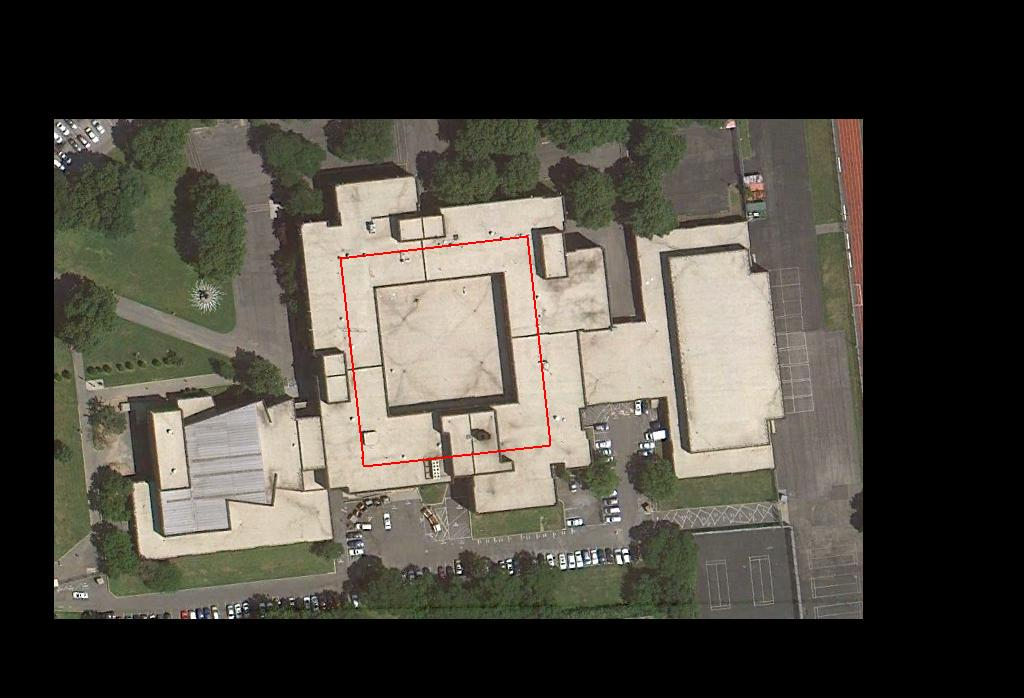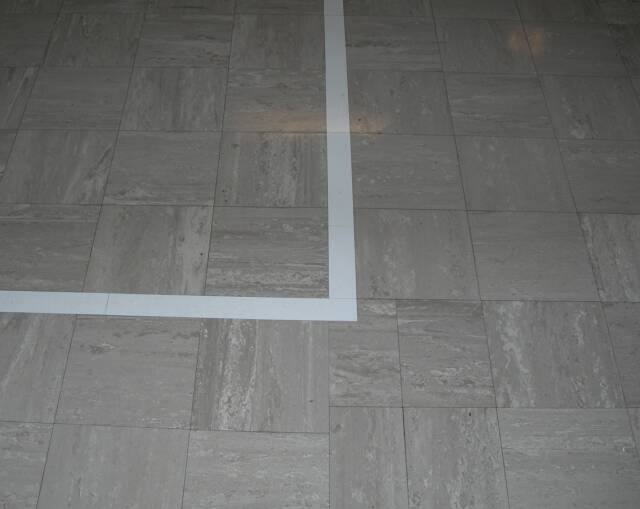Calculating the Average Speed
of a
John Dewey HS Physics Student

Interactive Laboratory Activity

Objective. The purpose of this lab is to determine the average rate of speed of a John Dewey HS student as he or she circumnavigates the third floor at his or her natural gait using the basic tools of distance and time measurement and a knowledge of basic kinematics.

Introduction. Using meter sticks and stopwatches the average speed of a John Dewey HS physics student can be determined by dividing the total distance traveled along a prescribed course by the total time of travel. Expressed mathematically,

vaverage = dtotal/ttotal

Materials.meter stickstopwatchcalculatorJohn Dewey HS physics students

Procedure. You and your lab group members must cooperatively collaborate on a strategy to determine each member's average speed as he or she circumnavigates the entire third floor. Each member must be reminded to walk at his or her normal gait along the white tile strip embedded into the floor along the center of each corridor and that the total distance walked is equivalent to the length of the strip which must in turn be measured by the group. The total time required for each group member to completely traverse the entire length of the strip (in seconds) as well as the total length of the strip (in meters) must be recorded. Dividing the measured length of the strip (dtotal) by the total time of each member's trip (ttotal) results in that person's average speed (vaverage) in meters per second.

Data and Data Analysis. Each member must record his or her as well as each and every other group member's ttotal and calculate his or her and every or group member's vtotal and to record this data. Each member will then be required to calculate the average speed of the group (vaverage/group):

vaverage/group = ( vaverage member sum)/(number of members)

Groups will then post their group average speeds on the front board so that each student will then be able to calculate a class average speed (vaverage/class)

vaverage/class = (vaverage/group)/(number of groups)

Once your group average speeds have been calculated, group coordinators are to display their group's average speed data. All work (including variables, equations, substitutions and units) must be shown in your final lab report. Similarly, all data must be displayed in tabular form in your final lab report. Answers to all questions listed below must be included in your final lab report

Questions.
1.  State your personal average speed. State your group's average speed. State the class average speed. How does your personal average speed compare to the class average speed?
2.   List the assumptions you and your group made in determining the distance around the third floor. List any potential sources of error that might have occurred as a result of the distance-measuring strategy you employed.
3.   Convert your average speed into kilometers per hour using dimensional analysis as well as your conversions/equivalences sheet. At your personal average speed, calculate the time it would take you to walk 1 kilometer. Show all work.
4.   Convert your average speed into miles per hour using dimensional analysis as well as your conversions/equivalences sheet. At your personal average speed, calculate the time it would take you to walk 1 mile.
5.   Calculate how far you can walk in (A)1 hour and (B) 5 minutes. Calculate your average speed in feet per second.
6.   Briefly summarize your group's distance-measuring strategy. Relate the mechanics of how the distances were physically measured. Explain how your group's average speed was derived.
7.   Explain why different people walk at different rates and how these differences affected the class average speed.

Acknowledgement. The original idea and design of this interactive laboratory activity sprang from the creative imagination of retired veteran Physics teacher Mr. Jack DePalma, formerly of Brooklyn Tech HS.Satellite Image of John Dewey High School
[Scale Sketch Map of Walking Path Overlain in Red]Room 353Walking PathWhite Tile Corridor Lane Line Intersection
[Outside Room 353]
White Tile
Corridor Lane
Line